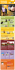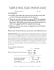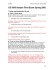# CmSc 150, Sample Test 1 1 Name: ________________________

## Transcription

CmSc 150, Sample Test 1 1 Name: ________________________
```CmSc 150, Sample Test 1
Page 1 of 7
Name: ________________________
1. (1 pt) Write a program that will print the name of a place you would like to win a
free trip to:
2. (1 pt) What does this program print?
# x=5
# print(x)
3. (1 pt) What does this program print?
x=5
print("x")
4. (3 pts) Write a program that asks the user to type in their first name and their
last name. Then print both the first and last name on the same line.
5. (1 pt) What does this print?
x=True
if x:
print ("Left")
else:
print ("Right")
CmSc 150, Sample Test 1
Page 2 of 7
6. (2 pts) Write a line of code that will take a price from the user.
* The number should be stored as a float, not a string.
7. (2 pts) Continuing from the prior question, calculate the amount of a tip,
assuming it is at 18%.
8. (2 pts) Continuing from the prior question, print the tip you calculated along with
a label (in dollars).
CmSc 150, Sample Test 1
9. (3 pts) Next to each section, list the number that it prints out:
# Section 1
x=5
x=x+5
print (x)
# Section 2
x=8
x+2
print(x+3)
# Section 3
x=5
x+2
print(x)
# Section 4
x=-10
x+=1
print(x)
# Section 5
x=6
x=x+4
print(x+1)
10. (2 pts) What does this code print?
my_text ="ABCD"
print ("The 1st spot is: "+ my_text )
print ("The -1 spot is: "+ my_text [-1])
Page 3 of 7
CmSc 150, Sample Test 1
Page 4 of 7
11. (2 pts) Cross out the variable names that are not legal in Python. (Note, this
question does not ask which variable names are proper.)
speed
circle width
speed2
square_length
speed2isTerrible
accountBalance
55isTooFast
accountBalance
Gigawatts
salesTax\$
12. (3 pts) Write a Python program that will take a number stored in the variable
grade and print if it is A ( ≥ 90), passing ( ≥ 60) or failing. Assume the variable
13. (1 pt) How do you know if you should create a “while” loop or a “for” loop?
CmSc 150, Sample Test 1
14. (3 pts) What does this code print?
a = 5<6
b = 6>=7
c = 5!=5
d = 5==15
print (a,b,c,d)
if a and b:
print ("A")
else:
print ("B")
15. (2 pts) Write a “for” loop that will print the numbers from 1 to 50 (inclusive).
16. (3 pts) Write a “while” loop that counts by 5’s from 0 up to 100 (inclusive).
Page 5 of 7
CmSc 150, Sample Test 1
Page 6 of 7
17. (2 pts) Given the list below, write code that will print the first item and the last
item.
my_list=[5,2,6,8]
18. (2 pts) Explain how colors are specified in Python when doing graphics.
19. (2 pts) Explain how coordinates for computer graphics differ from coordinates
normally used in graphing mathematical items.
CmSc 150, Sample Test 1
Page 7 of 7
20. (4 pts) Write code using two nested for loops that will output the following:
0
0
0
0
0
0
0
0
0
0
1
1
1
1
1
1
1
1
1
2
2
2
2
2
2
2
2
3
3
3
3
3
3
3
4
4
4
4
4
4
5
5
5
5
5
6
6 7
6 7 8
6 7 8 9
21. (4 pts) This code is to check a username/password combination. Find at least 4
things wrong with this code. (If the same mistake exists in more than one spot,
that only counts as one item.)
isAccessDenied=False
while isAccessDenied
if name = "Paul" or password="QBertsHammer":
print ("You may enter")
isAccessDenied=False
else:
```

### Exam 3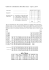### Automata Theory Sample Midterm Exam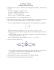### Math 0120 Examination #3 Sample Name (Print)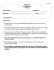### Math 30G – Calculus – Sample Final Exam Fall 2012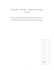### 3w 3 t5+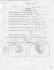### 2 for 1 Dinner Menu - Club Regent Casino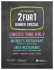### Cover Sheet! EXAM2 PRINT your name legibly on the line below.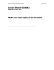### Elementary Algebra Sample Test #1 1. 2.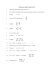### Byzantine ARt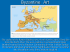### STAT 100 Midterm 1 Sample Exam - Spring 2013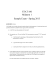### STEP OUT FOR A COLORFUL AUTUMN TREK!